# Waveguide Mathematics

Our other info on waveguide can be found on these pages:

Waveguide primer (main waveguide page)

Waveguide construction

Waveguide dimensions and letter bands

Waveguide loss

This page contains some of important equations for rectangular waveguide. Here is an index to the subject of waveguide mathematics:

Cutoff frequencies

Guide wavelength

Phase velocity and group velocity

Group delay in waveguide

Note that in the equations on this page we have kept the units simple and consistent, and you might want to do the same.... for time, use nanoseconds. For distance, use cm. For velocity, use cm/nanosecond. For frequency, use GHz.

### Cutoff frequencies

Waveguide can support many modes of transmission. All microwave textbooks will tell you about this, but we don't really care. The usual mode of transmission in rectangular waveguide is called TE10. Thanks for the correction, Jean-Jacques!) The upper cutoff wavelength (lower cutoff frequency) for this mode is very simply: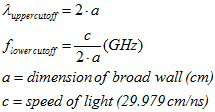The upper cutoff frequency is exactly one octave above the lower. We'll let you do the math on this (multiply lower cutoff frequency by two...) now it's time for another Microwaves101 rule of thumb:

###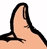Waveguide operating band

The accepted limits of operation for rectangular waveguide are (approximately) between 125% and 189% of the lower cutoff frequency. Thus for WR-90, the cutoff is 6.557 GHz, and the accepted band of operation is 8.2 to 12.4 GHz. Remember, at the lower cutoff the guide simply stops working. See our page on waveguide loss for more information.

### Guide wavelength

Guide wavelength is defined as the distance between two equal phase planes along the waveguide. The guide wavelength is a function of operating wavelength (or frequency) and the lower cutoff wavelength, and is always longer than the wavelength would be in free-space. Here's the equation for guide wavelength: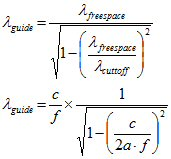Guide wavelength is used when you design distributed structures in waveguide. For example, if you are making a PIN diode switch with two shunt diodes spaces 3/4 wavelength apart, use the 3/4 of a guide wavelength in your design.

The guide wavelength in waveguide is longer than wavelength in free space. This isn't intuitive, it seems like the dielectric constant in waveguide must be less than unity for this to happen... don't think about this too hard you will get a headache.

Here is a way to imagine why this is... picture yourself at Zuma Beach in Malibu. The waves are coming in at an angle to the beach.... check out the intersection of the wavefront with the beach, it is zipping along faster than you can run... yes, it's apparently faster than the waves are moving if you look straight at them.New for December 2011! We now have a video of waves breaking sideways that illustrates phase velocity. Hopefully soon we will figure out how to embed it on this page for your enjoyment and education, stay tuned! Thanks to Michael!

Phase velocity and group velocity

Phase velocity is an almost useless piece of information you'll find in waveguide mathematics; here you multiply frequency times guide wavelength, and come up with a number that exceeds the speed of light!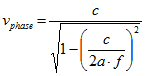Be assured that the energy in your wave is not exceeding the speed of light, because it travels at what is called the group velocity of the waveguide: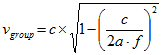The group velocity is always less than the speed of light, we like to think of that this is because the EM wave is ping-ponging back and forth as it travels down the guide. Note that group velocity x phase velocity = c2.

Group velocity in a waveguide is speed at which EM energy travels in the guide. Plotted below as a percentage of the speed of light (c), we see how group velocity varies across the band for WR-90 (X-band) waveguide. Note that the recommended operating band of WR-90 is from 8.2 to 12.4 GHz. At 8.2 GHz the signal is slowed to 60% of the free-space speed of light. At the lower cutoff (6.56 GHz), the wave is slowed to zero, and you can outrun it without breathing hard.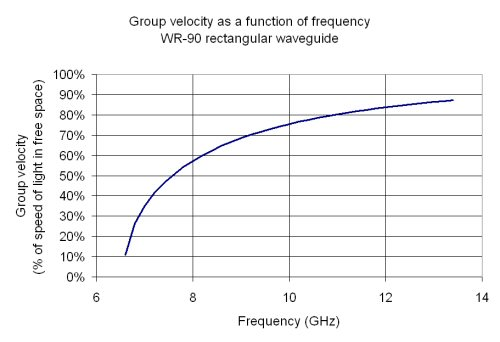### Group delay in waveguide

Now that we know the group velocity, we can calculate the group delay of any piece of waveguide, noting that time is distance divided by velocity: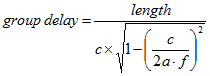The group delay of rectangular waveguide components is a function of the frequency you are applying. Near the lower cutoff, the group delay gets longer and longer, as the EM wave ping-pongs down the guide, and can easily be 10X the free-space group delay. But at the upper end of a waveguide's band, the group delay approaches the free-space group delay, which follows the rule-of-thumb, approximately one foot per nanosecond, independent of frequency.

To compare with the one nanosecond/foot rule of thumb, below is a plot of the group delay of one foot of WR-90 waveguide. At the upper end of the band you will see that very nearly the free-space group delay is achieved.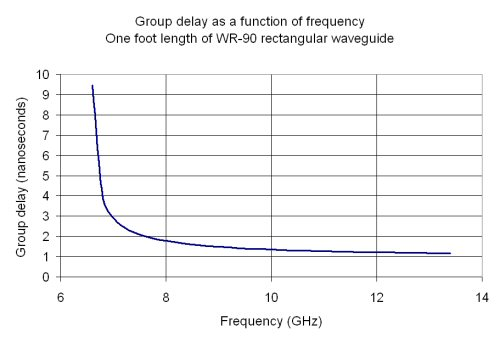The problem of electromagnetic energy traveling at different speeds over frequency is commonly called dispersion. Soon we will have a page on this topic as well.

Author : Unknown Editor